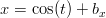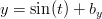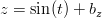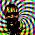## Saturday, January 16, 2010

### Chaotic Method of Manufactured Solutions: Lorenz 63

I quickly brushed over the method of manufactured solutions (MMS) aspects in the previous post on Lorenz 63, you might wonder why. Well, it turns out that applying the MMS to chaotic systems includes an additional consideration that is not normally of concern. Here is the ’standard’ sort of checklist for choosing manufactured solutions (taken from Verification of Computer Codes in Computational Science and Engineering, emphasis mine):

1. Manufactured solutions should be sufficiently smooth on the problem domain so that the theoretical order-of-accuracy can be matched by the observed order-of-accuracy obtained from the test. A manufactured solution that is not sufficiently smooth may decrease the observed order-of-accuracy (however, see # 7 in this list).
2. The solution should be general enough that it exercises every term in the governing equation. For example, one should not choose temperature T in the unsteady heat equation to be independent of time. If the governing equation contains spatial cross-derivatives, make sure the manufactured solution has a non-zero cross derivative.
3. The solution should have a sufficient number of nontrivial derivatives. For example, if the code that solves the heat conduction equation is second order in space, picking T in the heat equation to be a linear function of time and space will not provide a sufficient test because the discretization error would be zero (to within round-off) even on coarse grids.
4. Solution derivatives should be bounded by a small constant. This ensures that the solution is not a strongly varying function of space or time or both. If this guideline is not met, then one may not be able to demonstrate the required asymptotic order-of-accuracy using practical grid sizes. Usually, the free constants that are part of the manufactured solution can be selected to meet this guideline.
5. The manufactured solution should not prevent the code from running successfully to completion during testing. Robustness issues are not a part of code order verification. For example, if the code (explicitly or implicitly) assumes the solution is positive, make sure the manufactured solution is positive; or if the heat conduction code expects time units of seconds, do not give it a solution whose units are nanoseconds.
6. Manufactured solutions should be composed of simple analytical functions like polynomials, trigonometric, or exponential functions so that the exact solution can be conveniently and accurately computed. An exact solution composed of infinite series or integrals of functions with singularities is not convenient.
7. The solution should be constructed in such a manner that the differential operators in the governing equations make sense. For example, in the heat conduction equation, the flux is required to be differentiable. Therefore, if one desires to test the code for the case of discontinuous thermal conductivity, then the manufactured solution for temperature must be constructed in such a way that the flux is differentiable. The resultant manufactured solution for temperature is nondifferentiable.
8. Avoid manufactured solutions that grow exponentially in time to avoid confusion with numerical instability.

As emphasized in # 4 we often have to use the free constants in our manufactured solution to bound the derivatives in the domain. For chaotic systems we’ll need to use not only the free constants, but the initial conditions as well, to achieve another end: ensure that the chosen solution is in the convergent region at all times. This is a sufficient but not necessary condition for the source term to be in the basin of entrainment. When the source term is in the basin of entrainment then we can expect to be able to verify the ordered convergence of our implementation as we would in the non-chaotic case. Otherwises we can expect the truncation error to eventually lead to an arbitrarily large departure from the manufactured solution. This terminology comes from the nonlinear dynamics and control literature  . A worked example for the Lorenz ’63 system showing the boundary of the convergent region is in Chapter 6 of .

The convergent region is defined by the region of phase space for which the eigenvalues of the system Jacobian has negative real parts. As in any other application of the MMS, we start by choosing some convenient solution consistent with the desiderata enumerated above. Trigonometric functions are convenient in this case:(1)(2)(3)

Now we need the Jacobian of the system (and then its eigenvalues), since our governing equations are definied in Maxima, that is easy enough:

/* Need the Jacobian of the system to find the convergent region so
we can select a useful IC/amplitude for our manufactured solutions */
sys_eqns : [eqn_1, eqn_2, eqn_3] $J_cr : zeromatrix(3,3)$
for j : 1 thru 3 do
for i : 1 thru 3 do
(
J_cr[i,j] : diff(rhs(sys_eqns[i]), unknowns[j])
)
) $[J_cr_eigvals, J_cr_eigmult] : eigenvalues(J_cr)$
J_cr_eigvals : fullratsimp(J_cr_eigvals) 

As before these expressions are output in Fortran90 format for compilation. Here are the eigenvalues in the complex plane for bx = 1.0, by = -1.0, bz = 10.0.

 Figure 1: Jacobian eigenvalues for manufactured solution, bx = 1.0, by = -1.0, bz = 10.0

We can immediately see the problem from Figure 1: our manufactured solution is in the region of phase space where one of the Jacobian’s eigenvalues has a positive real part. We can adjust the initial conditions of our solution to get all of our eigenvalues on the left half-plane.

 Figure 2: Jacobian eigenvalues for manufactured solution, bx = 10.0, by = -10.0, bz = 40.0

Being limited to only certain portions of the phase space for conducting verification is fine, because a single well-designed manufactured solution verifies the correctness of the entire implementation (as long as all of the terms are activated). This is the same reason that it is often emphasized in the V&V literature that there is no need for the manufactured solution to be physically meaningful.

### References

   Jackson, E.A., “Controls of dynamic flows with attractors,” Physics Review A, Vol. 44, Is. 8, 1991.

   Wu, W., “Analytical and Numerical Methods Applied to Nonlinear Vessel Dynamics and Code Verification for Chaotic Systems,” Disertation, Virginia Polytechnic Institute and State University, Dec, 2009.

   W. Wu, L.S. McCue, and C.J. Roy. “The method of manufactured solutions applied to chaotic systems,” Nonlinear Dynamics, 2009, under review.

1.Hello

I am visiting here for the first time because Dan Hughes sent me a mail pointing to your blog .
First . My chaos interest comes from the mathematical physics point of view so I have difficulty to read and interpret the pages of code that you post .
If I understood well , you began to look at 2 aspects of deterministic chaos - convergence and stochasticity .
As it happens I have a rather good knowledge of both so if you continue being interested by the chaos theory , I could perhaps show you some avenues .

- The convergence question is easy .
There is none .
A fast qualitative argument bases on the Lyapounov coefficient . The size of the step used in the numerical solution can be considered as the difference between 2 initial conditions . But as any chaotic system has at least one positive Ltapounov coefficient , we know that 2 trajectories diverge exponentially .
Now the Heisenberg uncertainty relation gives us the lower bound for the accuracy with which we can distinguish 2 different initial conditions .
Follows that the greatest accuracy achievable corresponding to this lower bound leads to trajectories that still diverge (exponentially).
In other words a quantum fluctuation is enough to give an upper bound on the predictability time . Beyond that time no converging solution is computable even in principle .

2.- stochasticity . Obviously a chaotic system cannot be predicted deterministically be it numerically or otherwise (see above) . Therefore a legitimate question is whether there can be a statistical description of the trajectories . Or of the frequency of places that the trajectory will visit what is a similar question .
Well here the answer is : sometimes yes .
The key word is ergodicity and that's why it is (almost) impossible to study the chaos theory without studying the ergodic theory too .
Indeed an ergodic system fulfills the conditions of the ergodic theorem which says broadly that "the time average of a dynamical parameter taken along a trajectory converges to the phase space average weighted by a suitable probability density function when time goes against infinity" .
The ergodicity is the condition of existence because it guarantees that a pdf exists such as it permits to connect the phase space states to the temporal evolutions .
Not all chaotic systems are ergodic . F.ex colliding hard spheres are chaotic and ergodic while planetary orbits are chaotic and non ergodic . The use of ergodicity in the former leads to statistical thermodynamics which works very well . On the other hand the unpredictability of planetary orbits is both in the deterministic and the stochastical sense .

How does the climate fit in ?
Well it doesn't fit in . The chaos theory is mathematically a problem of non linear ODE while the climate (and weather) is a problem of non linear PDE . Space matters . We deal here with SPATIO-temporal chaos . The best way to intuitively grasp teh spatio temporal chaos is to look at the cigarette smoke . You clearly see that chaotic structures exist both in time and in space . The ergodic theorem applies only on dynamical systems depending on time . Finding an equivalent of ergodicity for spatio-temporal systems is cutting edge science - it is just beginning .
What is known and what the cigarette smoke also demonstrates is that while temporal chaos is often ergodic in physical systems of interest , it is not the case for spatio-temporal systems .
One consequence of that is that there is no equivalent of statistical thermodynamics for the Navier Stokes equations - you can't find "statistical" properties of solutions .
We don't even know if there is a unique smooth solution for given boundary/initial conditions . Kolmogorov turbulence theory is a kind of attempt at statistical fluid dynamics but it doesn't work well and it fails completely for lower Reynolds numbers .
So when one sees that in the so called "climatology" people get rid of the space by simply ... AVERAGING (!) and then "analyse" the time series of spatially averaged parameters (like GMST) , one wonders what kind of physics they do . Etc .
Well I think I have been too long already so I hope it shed some light .

3.Hi Tom,
Thank you for leaving such thoughtful comments. Dan emailed me too and gave me a 'nudge' about actually doing some demonstration calculations based on the ideas in this post: they are at the next post, which I think you'll find interesting.

You said: The size of the step used in the numerical solution can be considered as the difference between 2 initial conditions . But as any chaotic system has at least one positive Ltapounov coefficient , we know that 2 trajectories diverge exponentially .

I understand that, but my background is engineering, so I'm interested in the behaviour at finite time. So I'm happy as long as the time-step size or the numerical method still gives me a 'knob' I can tweak to get arbitrarily close to the solution for arbitrary (but finite) times. The convergence results in this post and this post seem to show that this is quite possible.

You said:Kolmogorov turbulence theory is a kind of attempt at statistical fluid dynamics but it doesn't work well and it fails completely for lower Reynolds numbers.

While that's true, it's also quite understandable considering the assumptions that go in to deriving the scaling relations of Kolmogorov's 'energy cascade', we shouldn't expect it to work at low Re.

You said: So when one sees that in the so called "climatology" people get rid of the space by simply ... AVERAGING (!) and then "analyse" the time series of spatially averaged parameters (like GMST) , one wonders what kind of physics they do .

I agree, boiling the climate down to a single globally averaged number is foolish, and most likely politically motivated. Averaging on smaller scales in space and time and introducing models for the extra terms you introduce is practically useful though (RANS / LES). I'm not really familiar with the internals of the climate models, do they do LES (wasn't Smagorinsky a climate modeler)?

4.Hello

Only fast today .
It is not for ANY finite time . There is an upper bound given by the Heisenberg relation . But even the Heisenberg accuracy/step is completely out of reach of any computer .
So what is practically feasible leads to very short times and if you wanted to go beyond , you'd need to decrease the steps exponentially .
That gives an upper time limit for numerical solutions very fast .
In short there is no converged numerical solution .
Actually you can not even be sure that a limit when the step goes to 0 is near to what you get with non 0 steps .
That's why some people call all these curves numerical artefacts because nobody knows if those curves are parts of solutions restricted to some finite [0,T] interval .
Not really surprising for a chaotic system where even the beginning may change when the values reach , pass some thresholds :)

I don't do numerical models so have little experience with climate models too . But they use LES in the dynamical core .
However one must not think that the climate models "solve" N-S in any sense of the word !
What they basically do is to compute 2 different equilibrium solutions and compare them .
It's 2 snapshots , it is not a movie .

5.I buy the upper-bound part, I kind of experienced that with this little toy problem, trying to converge the solutions further and further out required really cranking down the time-step size to the point that it became impractical (this is a blog after all, it's not worth waiting a week for solutions). And the ensemble where the ICs were perturbed on the order of the round-off error all eventually diverged (at about the same time) no matter what the time-step.

That's why some people call all these curves numerical artefacts because nobody knows if those curves are parts of solutions restricted to some finite [0,T] interval .

This is the part I have trouble with, if I can show that my implementation converges to a manufactured solution, and I can show time-step convergence for an ensemble (with IC's perturbed on the order of the machine precision), then haven't I shown that the trajectory is not a numerical artifact?

I haven't done much reading on 'chaos' per se, do you have any references you could point me at that would clear this up (no hurry, this is just my idle curiosity)?

6.Interesting discussion. My thanks to both of you.

7.Ok, I thought about it some more; I guess you could argue that even if you put your ensemble member's ICs as close as possible given the limits of your machine, there could still be ICs 'between' those that are in different basins of attraction and you'd never know it.

8.Since Tom got me thinking, I found this article on attractors which discusses the effect of uncertainty in initial conditions has on determining which basin an IC is in. Basins can have fractal boundaries, so it could have 'interesting' features even at the scale of the machine precision.

9.Well you are rediscovering the chaos theory ;)
I already mentioned it but would like to stress again the importance of ergodicity .
The Lorenz system is ergodic that's why eventual results obtained with this system can't be generalized to all chaotic systems that might be non ergodic .
Ergodicity means broadly that the trajectories go everywhere in the attractor . In other words with any initial conditions if you wait long enough the trajectory will visit all points .
Hence the notion of pdf and the possibility to treat the system stochastically (of course the individual trajectories can't still be predicted beyond some finite time) .

10.But if the system is NOT ergodic , it means that there are "holes" and "traps" . For such system you can be never sure what it will do for a given initial condition . Even 2 initial conditions separated by the smallest possible interval (the Heisenberg limit) might lead to 2 very different trajectories .
You are right that fractal sets (strange attractors) are typically an example .
I will take an example of convergence difficulty - the Newton-Rhapson iteration .
If you apply it to some complex polynomial (f.ex z^4 - 1) and vary the initial conditions you will obtain a fractal boundary between the points that lead to a convergence to one of the 4 roots .
That means that there will be an infinity of points such as that you can make an interval I as small as you want around these points and for points interior to this interval you will converge to any of the 4 roots .
So actually you are unable to say to what root will converge the Newton Raphson iteration for a given initial point regardless of the smallness of the interval between 2 initial conditions .

The Lorenz attractor is a "well behaved" chaos .
While the attractor itself is strange , its strangeness only translates the already known "banal" chaos feature - unpredictability of individual trajectories .
But its boundary is not fractal so you will always finish on the attractor if you are in the chaotic regime .
Once you are on it , the ergodicity will make sure that you will run through it by visiting every part but the trajectories will NEVER intersect (demonstrated very important result for all chaotic systems) .
This property allows me to mention an amusing paradox showing the problems with numerical integrations .
You choose the smallest possible integration step that the best computer allows .
You take some initial condition and begin to compute . Given the computer accuracy there is a FINITE number of different points it can represent .
Even if this number is very large , if you compute long enough your trajectory will eventually find a point which is EXACTLY the same that it has already visited and from that point on your computer will only periodically cycle this same trajectory .
The computer just violated in the major way the fundamental rule that trajectories never intersect !
And I have just proven that it will always happen with any computer with finite accuracy :)

As for the books , there are of course many of varying difficulty .
Personnaly I like and usually recommend the R.Hilborn's Introduction to chaos and non linear dynamics . Comprehensive , rigorous and gives all the references and ways to go deeper in the matters if one wishes to do so .

11.You gents might appreciate the discussion over here on this paper about the effects of different hardware / software on ensemble results.

From the abstract:
[...] We demonstrate that effects of parameter, hardware and software variation are detectable, complex and interacting. However we find most of the effects of parameter variation are due to a small subset of parameters. [...] We demonstrate the effect of hardware and software is small relative to the effect of parameter variation and, over the wide range of systems tested, may be treated as equivalent to that caused by changes in initial conditions.

12.Interesting comment on control of chaotic systems, which is very closely related to verification with MMS (since control terms show up as forcings in the governing equations).

13.The point Tomas raises here about averages being chaotic deserves a post in the Lorenz63 series; that's something we can show with the toy model.